How Unit Generator plug-ins work.

The server loads unit generator plug-ins when it starts up.

Unit Generator plug-ins are dynamically loaded libraries (DLLs) written in C++.

Each library may contain one or multiple unit generator definitions.

The server looks in the "plugins" directory for all files ending in .scx and calls the load() function in each one.

When the library is loaded the server calls the load() function in the library.

The load function has two responsibilities:

• It needs to store the passed in pointer to the InterfaceTable in a global variable.

• It defines the unit generators.

// InterfaceTable contains pointers to functions in the host (server).

static InterfaceTable *ft;

...

// the load function is called by the host when the plug-in is loaded

{

ft = inTable; // store pointer to InterfaceTable

DefineSimpleUnit(MySaw);

}

Unit Generators are defined by calling a function in the InterfaceTable and passing it the name of the unit generator, the size of its C data struct, and pointers to functions for constructing and destructing it. The macro DefineSimpleUnit makes this more brief.

#define DefineSimpleUnit(name) \

(*ft->fDefineUnit)(#name, sizeof(name), (UnitCtorFunc)&name##_Ctor, 0);

ft->fDefineUnit is a function pointer in the InterfaceTable to the server function that defines a new unit generator.

#name creates a string C from the name. In this case, "MySaw".

sizeof(name) will be the size of the struct MySaw.

name##_Ctor will macro-expand to MySaw_Ctor. There will need to be a function defined with this name.

0 is the argument for the Dtor, or destructor function, which is not needed for this unit generator.

So the macro:

DefineSimpleUnit(MySaw);

expands to this:

(*ft->fDefineUnit)("MySaw", sizeof(MySaw), (UnitCtorFunc)&MySaw_Ctor, 0);

A plug-in can also define things other than unit generators such as buffer fill ("/b_gen") commands.

You will need to have the Developer Tools installed to do this.

Each group of plugins shares a target in Xcode, which outputs a single plugin, e.g MyUGens.scx.

We will use an existing target as a template for our target. In this tutorial we will use ChaosUGens as a template, although you could use any target.

The frist step is to make a duplicate of ChaosUGens. To do this control-click on the ChaosUGens target and select "Duplicate".Now we need to change the name of the target and it's output file. Double click on the "ChaosUGens copy" to open it's inspector.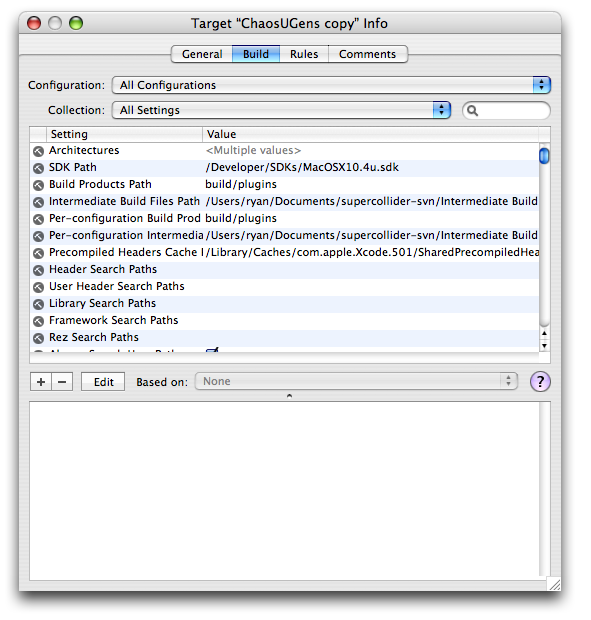In the "Build" tab , search for the "Product Name" setting and change the value to "MyUGens".Now switch to the "General" tab and change the target name to "MyUGens".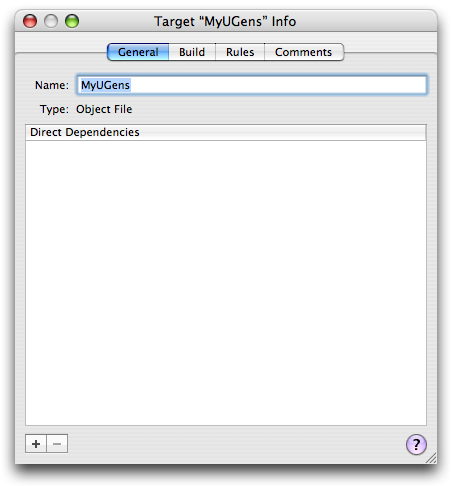Close the ChaosUGens inspector, expand the target's disclosure triangle, and then expand the "Compile Sources" disclosure triangle.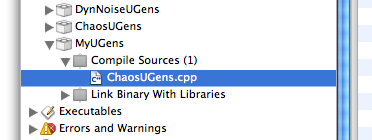Delete the existing "ChaosUGens.cpp" source file by clicking on it and pressing the delete key. Confirm the deletion in the dialog that is presented.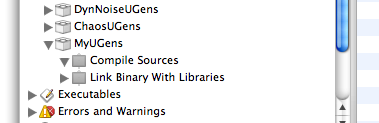Use the "File->New File..." menu option to create a new source file.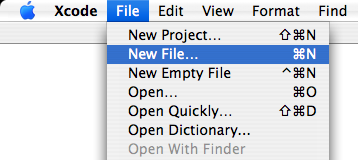Under the BSD section, select "C++ File", and click "Next".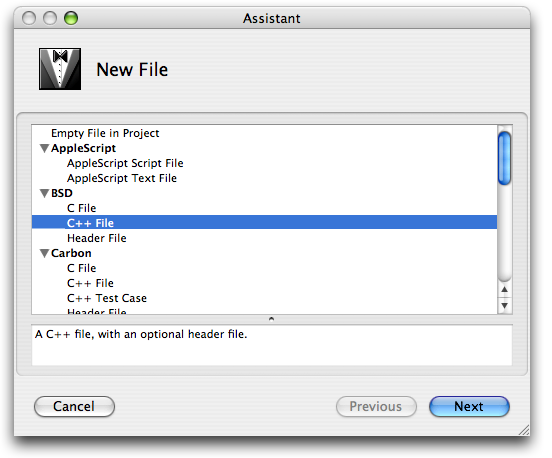Change the filename field to "MyUGens.cpp", uncheck the "Also create MyUGens.h" option, and check "MyUGens" as the only target. When all these options have been set, click "Finish".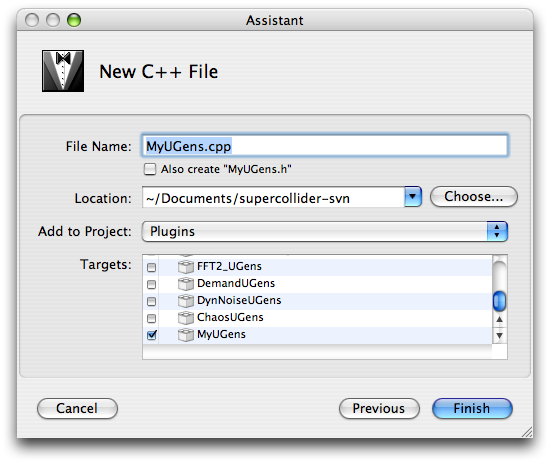Erase the default code, and copy the following into the MyUGens.cpp file:

____________________________________________________________________

#include "SC_PlugIn.h"

// InterfaceTable contains pointers to functions in the host (server).

static InterfaceTable *ft;

// declare struct to hold unit generator state

struct MySaw : public Unit

{

double mPhase; // phase of the oscillator, from -1 to 1.

float mFreqMul; // a constant for multiplying frequency

};

// declare unit generator functions

extern "C"

{

void MySaw_next_a(MySaw *unit, int inNumSamples);

void MySaw_next_k(MySaw *unit, int inNumSamples);

void MySaw_Ctor(MySaw* unit);

};

//////////////////////////////////////////////////////////////////

// Ctor is called to initialize the unit generator.

// It only executes once.

// A Ctor usually does 3 things.

// 1. set the calculation function.

// 2. initialize the unit generator state variables.

// 3. calculate one sample of output.

void MySaw_Ctor(MySaw* unit)

{

// 1. set the calculation function.

if (INRATE(0) == calc_FullRate) {

// if the frequency argument is audio rate

SETCALC(MySaw_next_a);

} else {

// if the frequency argument is control rate (or a scalar).

SETCALC(MySaw_next_k);

}

// 2. initialize the unit generator state variables.

// initialize a constant for multiplying the frequency

unit->mFreqMul = 2.0 * SAMPLEDUR;

// get initial phase of oscillator

unit->mPhase = IN0(1);

// 3. calculate one sample of output.

MySaw_next_k(unit, 1);

}

//////////////////////////////////////////////////////////////////

// The calculation function executes once per control period

// which is typically 64 samples.

// calculation function for an audio rate frequency argument

void MySaw_next_a(MySaw *unit, int inNumSamples)

{

// get the pointer to the output buffer

float *out = OUT(0);

// get the pointer to the input buffer

float *freq = IN(0);

// get phase and freqmul constant from struct and store it in a

// local variable.

// The optimizer will cause them to be loaded it into a register.

float freqmul = unit->mFreqMul;

double phase = unit->mPhase;

// perform a loop for the number of samples in the control period.

// If this unit is audio rate then inNumSamples will be 64 or whatever

// the block size is. If this unit is control rate then inNumSamples will

// be 1.

for (int i=0; i < inNumSamples; ++i)

{

// out must be written last for in place operation

float z = phase;

phase += freq[i] * freqmul;

// these if statements wrap the phase a +1 or -1.

if (phase >= 1.f) phase -= 2.f;

else if (phase <= -1.f) phase += 2.f;

// write the output

out[i] = z;

}

// store the phase back to the struct

unit->mPhase = phase;

}

//////////////////////////////////////////////////////////////////

// calculation function for a control rate frequency argument

void MySaw_next_k(MySaw *unit, int inNumSamples)

{

// get the pointer to the output buffer

float *out = OUT(0);

// freq is control rate, so calculate it once.

float freq = IN0(0) * unit->mFreqMul;

// get phase from struct and store it in a local variable.

// The optimizer will cause it to be loaded it into a register.

double phase = unit->mPhase;

// since the frequency is not changing then we can simplify the loops

// by separating the cases of positive or negative frequencies.

// This will make them run faster because there is less code inside the loop.

if (freq >= 0.f) {

// positive frequencies

for (int i=0; i < inNumSamples; ++i)

{

out[i] = phase;

phase += freq;

if (phase >= 1.f) phase -= 2.f;

}

} else {

// negative frequencies

for (int i=0; i < inNumSamples; ++i)

{

out[i] = phase;

phase += freq;

if (phase <= -1.f) phase += 2.f;

}

}

// store the phase back to the struct

unit->mPhase = phase;

}

////////////////////////////////////////////////////////////////////

// the load function is called by the host when the plug-in is loaded

{

ft = inTable;

DefineSimpleUnit(MySaw);

}

////////////////////////////////////////////////////////////////////

____________________________________________________________________

Drag the MyUGens target into the "All" aggregate target, and build the project using the "Build->Build" menu option.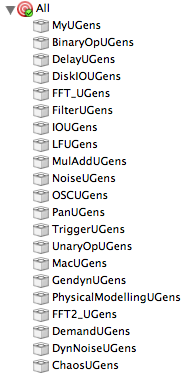Now launch SuperCollider.app and create a file named MyUGens.sc in the class library. Add the following to this file:

____________________________________________________________________

MySaw : UGen {

*ar { arg freq = 440.0, iphase = 0.0, mul = 1.0, add = 0.0;

}

*kr { arg freq = 440.0, iphase = 0.0, mul = 1.0, add = 0.0;

}

}

____________________________________________________________________

The SuperCollider class for your UGen allows the SuperCollider application to be able to write a SynthDef file.

The arguments to the MySaw UGen are freq and iphase.

The multiNew method handles multi channel expansion.

The madd method provides support for the mul and add arguments. It will create a MulAdd UGen if necessary. You could write the class without mul and add arguments, but providing them makes it more convenient for the user.

MySaw : UGen {

*ar { arg freq = 440.0, iphase = 0.0;

^this.multiNew('audio', freq, iphase)

}

*kr { arg freq = 440.0, iphase = 0.0;

^this.multiNew('control', freq, iphase)

}

}

____________________________________________________________________

After a recompilation of the class library (Menu: Lang > Complie Library), the UGen is

// test it:

{ MySaw.ar(200,0,0.1) }.play

_________________________________________________________

Workflow

When changing the C-sourcecode, after each rebuilding the project, there is no need to restart SuperCollider or recompile the library (unless you have changed the class definition)

// just reboot the server.

s.reboot;

Note that if you want to use the internal server (e.g. for scoping), you do need to restart

the SuperCollider application.

_________________________________________________________

Useful macros

These are defined in SC_Unit.h.

// These return float* pointers to input and output buffers.

#define IN(index)  (unit->mInBuf[index])

#define OUT(index) (unit->mOutBuf[index])

// These return a float value. Used for control rate inputs and outputs.

#define IN0(index)  (IN(index))

#define OUT0(index) (OUT(index))

// get the rate of the input.

#define INRATE(index) (unit->mInput[index]->mCalcRate)

The possible rates are:

calc_ScalarRate

calc_BufRate "control rate"

calc_FullRate "audio rate"

// set the calculation function

#define SETCALC(func) (unit->mCalcFunc = (UnitCalcFunc)&func)

SETCALC must be called in the constructor. It may also be called from a calculation function to change to a different calculation function.

// calculate a slope for control rate interpolation to audio rate.

#define CALCSLOPE(next,prev) ((next - prev) * unit->mRate->mSlopeFactor)

CALCSLOPE returns (next - prev) / blocksize which is useful for calculating slopes for linear interpolation.

#define SAMPLERATE (unit->mRate->mSampleRate)

SAMPLERATE returns the sample rate for the unit generator. If it is audio rate then it will be the audio sample rate. If the ugen is control rate, then it will be the control rate. For example, if the ugen is control rate and the auio sample rate is 44100 and the block size is 64, then this will return 44100/64 or 689.0625.

#define SAMPLEDUR (unit->mRate->mSampleDur)

SAMPLEDUR is simply the reciprocal of the sample rate. It is the seconds per sample.

#define BUFLENGTH (unit->mBufLength)

BUFLENGTH is equal to the block size if the unit is audio rate and is equal to 1 if the unit is control rate.

#define BUFRATE (unit->mRate->mBufRate)

BUFRATE always returns the control rate.

#define BUFDUR (unit->mRate->mBufDuration)

BUFDUR is the reciprocal of the control rate.

____________________________________________________________________

Pointer aliasing

The server uses a "buffer coloring" algorithm to minimize use of buffers to optimize cache performance. This means that any of the output buffers may be the same as one of the input buffers. This allows for in-place operation which is very efficient. You must be careful however not to write any output sample before you have read all of the input samples. If you did, then the input will be overwritten with output.

// This code is correct. It reads the freq input before writing to out.

for (int i=0; i < inNumSamples; ++i)

{

float z = phase; // store phase in z

phase += freq[i] * freqmul; // read freq

out[i] = z; // write the output

// these if statements wrap the phase a +1 or -1.

if (phase >= 1.f) phase -= 2.f;

else if (phase <= -1.f) phase += 2.f;

}

// If out and freq are the same, then the code below will fail.

for (int i=0; i < inNumSamples; ++i)

{

// write the output

out[i] = phase;

phase += freq[i] * freqmul;

// these if statements wrap the phase a +1 or -1.

if (phase >= 1.f) phase -= 2.f;

else if (phase <= -1.f) phase += 2.f;

}

If your unit generator cannot be written efficiently when pointers are aliased, then you can tell the server this by using one of the following macros when definining it.

DefineSimpleCantAliasUnit(MyUGen);

DefineDtorCantAliasUnit(MyUGen);

The server will then ensure that no output buffers are the same as any input buffers.

____________________________________________________________________

A Unit Generator that needs a Dtor

This is code for a simple fixed delay line.

#include "SC_PlugIn.h"

// InterfaceTable contains pointers to functions in the host (server).

static InterfaceTable *ft;

// declare struct to hold unit generator state

struct MyDelay : public Unit

{

uint32 mDelayLength;

uint32 mPosition;

float *mData; // delay buffer

};

// declare unit generator functions

extern "C"

{

void MyDelay_next_notfull(MyDelay *unit, int inNumSamples);

void MyDelay_next_full(MyDelay *unit, int inNumSamples);

void MyDelay_Ctor(MyDelay* unit);

void MyDelay_Dtor(MyDelay* unit);

};

//////////////////////////////////////////////////////////////////

// Ctor is called to initialize the unit generator.

// It only executes once.

// A Ctor usually does 3 things.

// 1. set the calculation function.

// 2. initialize the unit generator state variables.

// 3. calculate one sample of output.

void MyDelay_Ctor(MyDelay* unit)

{

// 1. set the calculation function.

SETCALC(MyDelay_next_notfull);

// 2. initialize the unit generator state variables.

// get the delay length

unit->mDelayLength = (uint32)(IN0(1) * SAMPLERATE);

// allocate the buffer

unit->mData = (float*)RTAlloc(unit->mWorld, unit->mDelayLength * sizeof(float));

// RTAlloc allocates out of the real time memory pool of the server

// which is finite. Note that this memory is not zeroed, so it may contain

// garbage. Make sure to write before read.

// The size of the real time memory pool is set using the

// -m command line argument of the server, or by ways of server.options.memSize

// initialize the position

unit->mPosition = 0;

// 3. calculate one sample of output.

MyDelay_next_notfull(unit, 1);

}

//////////////////////////////////////////////////////////////////

// Dtor is called to perform any clean up for the unit generator.

void MyDelay_Dtor(MyDelay* unit)

{

// free the buffer

RTFree(unit->mWorld, unit->mData);

}

//////////////////////////////////////////////////////////////////

// The calculation function executes once per control period

// which is typically 64 samples.

// calculation function when the buffer has not yet been filled

void MyDelay_next_notfull(MyDelay *unit, int inNumSamples)

{

// get the pointer to the output buffer

float *out = OUT(0);

// get the pointer to the input buffer

float *in = IN(0);

// get values from struct and store them in local variables.

// The optimizer will cause them to be loaded it into a register.

float *data = unit->mData;

uint32 length = unit->mDelayLength;

uint32 position = unit->mPosition;

bool wrapped = false;

// perform a loop for the number of samples in the control period.

// If this unit is audio rate then inNumSamples will be 64 or whatever

// the block size is. If this unit is control rate then inNumSamples will

// be 1.

for (int i=0; i < inNumSamples; ++i)

{

// get old value in delay line

float z = data[position];

// store new value in delay line

data[position] = in[i];

// see if the position went to the end of the buffer

if (++position >= length) {

position = 0; // go back to beginning

wrapped = true; // indicate we have wrapped.

// change the calculation function

// next time, the MyDelay_next_full function will be called

SETCALC(MyDelay_next_full);

}

// if we have not yet wrapped, then z is garbage from the uninitialized

// buffer, so output zero. If we have wrapped, then z is a good value.

out[i] = wrapped ? z : 0.f;

}

// store the position back to the struct

unit->mPosition = position;

}

//////////////////////////////////////////////////////////////////

// calculation function when the buffer has been filled

void MyDelay_next_full(MyDelay *unit, int inNumSamples)

{

// get the pointer to the output buffer

float *out = OUT(0);

// get the pointer to the input buffer

float *in = IN(0);

// get values from struct and store them in local variables.

// The optimizer will cause them to be loaded it into a register.

float *data = unit->mData;

uint32 length = unit->mDelayLength;

uint32 position = unit->mPosition;

// perform a loop for the number of samples in the control period.

// If this unit is audio rate then inNumSamples will be 64 or whatever

// the block size is. If this unit is control rate then inNumSamples will

// be 1.

for (int i=0; i < inNumSamples; ++i)

{

// get old value in delay line

float z = data[position];

// store new value in delay line

data[position] = in[i];

// see if the position went to the end of the buffer

if (++position >= length) {

position = 0; // go back to beginning

}

out[i] = z;

}

// store the position back to the struct

unit->mPosition = position;

}

////////////////////////////////////////////////////////////////////

// the load function is called by the host when the plug-in is loaded

{

ft = inTable;

DefineDtorUnit(MyDelay);

}

////////////////////////////////////////////////////////////////////

____________________________________________________________________

// In the MyUGens.sc file:

MyDelay : UGen {

*ar { arg in, delaytime=0.4;

^this.multiNew('audio', in, delaytime)

}

*kr { arg in, delaytime=0.4;

^this.multiNew('control', in, delaytime)

}

}

____________________________________________________________________

// test it

(

{

var z;

z = SinOsc.ar * Decay.kr(Impulse.kr(1,0,0.2), 0.1);

[z, MyDelay.ar(z, 0.3)]

}.play;

)

____________________________________________________________________

TO DO:

UGens which access buffers.

UGens which use the built in random number generators.